## 5.5.1 The Exploration Algorithm

Before explaining how to use these trees to solve a planning query, imagine that the goal is to get as close as possible to every configuration, starting from an initial configuration. The method works for any dense sequence. Once again, let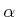denote an infinite, dense sequence of samples in. Theth sample is denoted by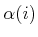. This may possibly include a uniform, random sequence, which is only dense with probability one. Random sequences that induce a nonuniform bias are also acceptable, as long as they are dense with probability one.

An RDT is a topological graph,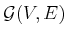. Let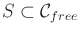indicate the set of all points reached by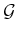. Since each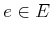is a path, this can be expressed as the swath,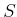, of the graph, which is defined as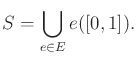(5.40)

In (5.40),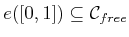is the image of the path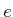.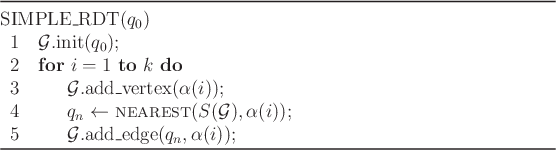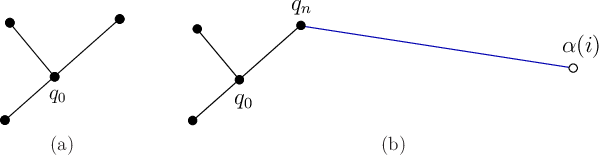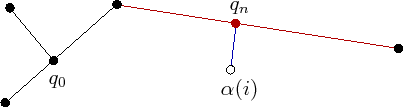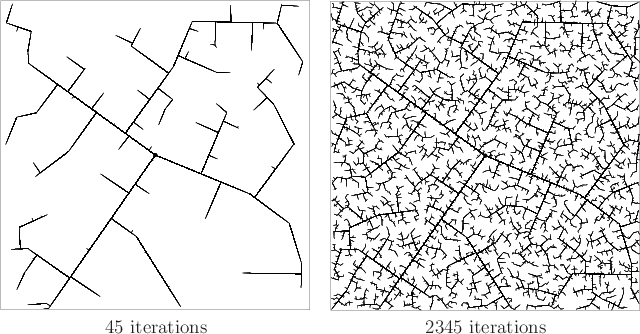The exploration algorithm is first explained in Figure 5.16 without any obstacles or boundary obstructions. It is assumed thatis a metric space. Initially, a vertex is made at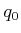. Foriterations, a tree is iteratively grown by connectingto its nearest point in the swath,. The connection is usually made along the shortest possible path. In every iteration,becomes a vertex. Therefore, the resulting tree is dense. Figures 5.17-5.18 illustrate an iteration graphically. Suppose the tree has three edges and four vertices, as shown in Figure 5.17a. If the nearest point,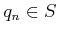, tois a vertex, as shown in Figure 5.17b, then an edge is made from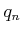to. However, if the nearest point lies in the interior of an edge, as shown in Figure 5.18, then the existing edge is split so thatappears as a new vertex, and an edge is made fromto. The edge splitting, if required, is assumed to be handled in line 4 by the method that adds edges. Note that the total number of edges may increase by one or two in each iteration.

The method as described here does not fit precisely under the general framework from Section 5.4.1; however, with the modifications suggested in Section 5.5.2, it can be adapted to fit. In the RDT formulation, the NEAREST function serves the purpose of the VSM, but in the RDT, a point may be selected from anywhere in the swath of the graph. The VSM can be generalized to a swath-point selection method, SSM. This generalization will be used in Section 14.3.4. The LPM tries to connecttoalong the shortest path possible in.

Figure 5.19 shows an execution of the algorithm in Figure 5.16 for the case in which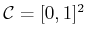and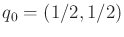. It exhibits a kind of fractal behavior.5.15Several main branches are first constructed as it rapidly reaches the far corners of the space. Gradually, more and more area is filled in by smaller branches. From the pictures, it is clear that in the limit, the tree densely fills the space. Thus, it can be seen that the tree gradually improves the resolution (or dispersion) as the iterations continue. This behavior turns out to be ideal for sampling-based motion planning.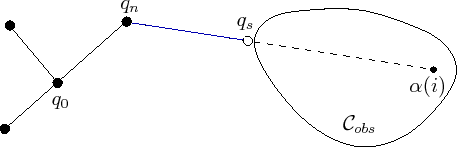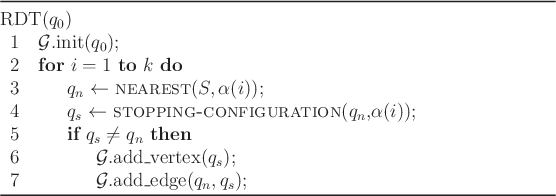Recall that in sampling-based motion planning, the obstacle region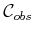is not explicitly represented. Therefore, it must be taken into account in the construction of the tree. Figure 5.20 indicates how to modify the algorithm in Figure 5.16 so that collision checking is taken into account. The modified algorithm appears in Figure 5.21. The procedure STOPPING-CONFIGURATION yields the nearest configuration possible to the boundary of, along the direction toward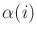. The nearest pointis defined to be same (obstacles are ignored); however, the new edge might not reach to. In this case, an edge is made fromto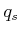, the last point possible before hitting the obstacle. How close can the edge come to the obstacle boundary? This depends on the method used to check for collision, as explained in Section 5.3.4. It is sometimes possible thatis already as close as possible to the boundary ofin the direction of. In this case, no new edge or vertex is added that for that iteration.

Steven M LaValle 2020-08-14